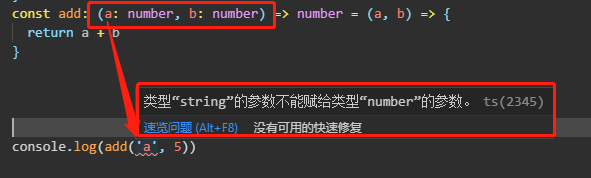«
typescript中的类型及定义

typescript 是一门静态类型语言

null, symbol, undefind, boolean, void

```// 首先定义一个Number类型的变量
let count: number = 12;
// String类型的变量
let name: string = 'xiaozhang'
// 注意这里编辑器会报错, 因为name是typescript保留的关键字```

`class Person {}`

```const person : {
name: string
age: number
} = {
name: '小张一号',
age: 18
};```

`const numbers: numbers[] = [1,2,3];`

```const add: (a: number, b: number) => number = (a, b) => {
return a + b
}```

`const xiaozhang: Person = new Person();`